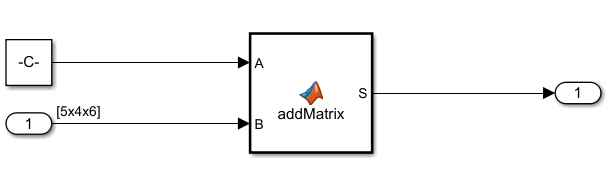## Generate Row-Major Code for Model That Contains a MATLAB Function Block

Programming languages and environments assume a single array layout for all data. MATLAB® and Fortran use column-major layout by default, whereas C and C++ use row-major layout. With Simulink® Coder™, you can generate C/C++ code that uses row-major layout or column-major layout.

MATLAB Function blocks enable you to define custom functionality in Simulink models by using the MATLAB language. You can generate row-major code for models that contain a MATLAB Function block by using row-major or column-major data. For more information on MATLAB Function blocks, see Implement MATLAB Functions in Simulink with MATLAB Function Blocks.

By default, the code generator generates column-major code. For C/C++ code generation, you can specify the array layout at the model level by using the Array layout model configuration parameter. Setting this parameter to `Row-major` enables the model for row-major code generation. To enable the MATLAB Function block in your model for row-major code generation, use the `coder.rowMajor` function at the function level inside the block.

### Row-Major Code Generation

For certain algorithms, row-major layout provides more efficient memory access. You get efficient code when you generate code for a model that uses row-major array layout and the model contains a MATLAB Function block that uses an algorithm for row-major data.

1. Consider an example model `ex_row_major_MLFB`.This model contains a Constant block that has a `[5 4]` matrix. To specify the matrix, set Constant value to:

`reshape(1:20,5,4)`
The Inport block also specifies a `[5 4]` matrix. To specify the matrix, set the Port dimensions to `[5 4]`.

2. In the Configuration Parameters dialog box, set Array layout to `Row-major`.

3. Write a function for matrix addition called `addMatrix`. The MATLAB Function block inherits the array layout setting from the model configuration parameter Array layout unless specified otherwise.

Optionally, you can use `coder.rowMajor` to explicitly set the array layout of the MATLAB Function block to row-major layout.

```function S = addMatrix(A,B) S = zeros(size(A)); for row = 1:size(A,1) for col = 1:size(A,2) S(row,col) = A(row,col) + B(row,col); end end```

4. Generate code for the model. From the C Code tab, click Build.

The code generator produces this C code:

```for (b_row = 0; b_row < 5; b_row++) { for (b_col = 0; b_col < 4; b_col++) { rtb_S_tmp = (b_row << 2) + b_col; rtb_S[rtb_S_tmp] = ex_row_major_MLFB_P.Constant_Value[rtb_S_tmp] + ex_row_major_MLFB_U.Inport1[rtb_S_tmp]; } }```
The generated code has two `for` loops. The first `for` loop accesses the rows and the second `for` loop accesses the columns. When the array layout of the MATLAB Function block and the model is the same, the generated code is efficient because no transposes or conversion are required.

### Mixed-Majority Code Generation

You can generate mixed-majority code when you have a model that operates on row-major data and a MATLAB Function block that operates on column-major data. When you generate code for a model that uses column-major layout, and the model contains a MATLAB Function block that uses row-major layout, then the code generator converts the block input data to row-major and the block output data back to column-major data, as needed. You can also generate mixed majority code when you have a model that operates on column-major data and a MATLAB Function block that operates on row-major data.

Array layout conversions can affect performance.

1. Consider the example model `ex_row_major_MLFB`. For more information on the example model, see Row-Major Code Generation.

In the Configuration Parameters dialog box, set Array layout to `Row-major`.

2. Update the `addMatrix` function in the MATLAB Function block for column-major data by using the `coder.columnMajor` function.

```function S = addMatrix(A,B) coder.columnMajor; S = zeros(size(A)); for row = 1:size(A,1) for col = 1:size(A,2) S(row,col) = A(row,col) + B(row,col); end end```
You can generate mixed-majority code by using the MATLAB Function block. In this case, you configure the model for row-major array layout and the MATLAB Function block for column-major array layout.

3. Generate code for the model. From the C Code tab, click Build.

The code generator produces this C code:

```for (b_row = 0; b_row < 4; b_row++) { for (b_col = 0; b_col < 5; b_col++) { B_tmp = (b_col << 2) + b_row; B_tmp_0 = b_col + 5 * b_row; B[B_tmp_0] = ex_row_major_MLFB_19b_U.Inport1[B_tmp]; A[B_tmp_0] = ex_row_major_MLFB_19b_P.Constant_Value[B_tmp]; } } for (b_row = 0; b_row < 5; b_row++) { /* Outport: '<Root>/Outport' */ for (b_col = 0; b_col < 4; b_col++) { B_tmp = 5 * b_col + b_row; ex_row_major_MLFB_19b_Y.Outport[b_col + (b_row << 2)] = A[B_tmp] + B[B_tmp]; }```
The inputs to the MATLAB Function block exist in a row-major environment. The code generator performs a conversion operation on inputs before they are fed to the MATLAB Function block because the block is column-major layout. After processing the algorithm in the MATLAB Function block, the code generator converts the data back to row-major data before passing the data to an Outport.